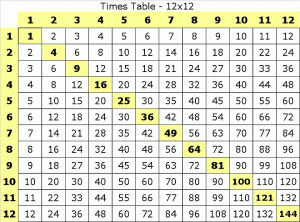# Times Tables DetectivesWhat patterns can you see on this times tables grid? What do you notice? Are any numbers on there more than once? Are any numbers not on the grid?

Let us know what you spot!

### 7 thoughts on “Times Tables Detectives”

•24th September 2020 at 8:20 am

I notice that the numbers in yellow(the ones that are diagonal)are squared numbers. So, like 6×6 is squared so the answer is 36:
1,4,9,16,25,36,49,64,81,100,121,144.

•27th September 2020 at 4:44 pm

How many times does each square number appear on the grid? What do you notice?

•24th September 2020 at 3:02 pm

Hi!
I notice that this grid goes up to 12X12.
I notice that this grid goes from 1 to 144.
I notice that this grid is a multiplication grid.
I notice that this grid is symetrycal.
I have to swap the laptops so I cannot do any more.

•24th September 2020 at 5:32 pm

I notice that the digits are only on there twice
I notice that it is 1 to 144
I think it is a square numbers

•24th September 2020 at 5:43 pm

I notice it is a square number
I notice it is 1 to 144
I notice that the numbers are on the grid twice
I notice that this grid is a multiplication grid

•30th September 2020 at 7:13 pm

The times tables go up to 1x to 12x .
i notice that there is a column of the times tables.
Also every number in any times table is highlighted.

•